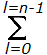# Electronics and Communication Engineering - Materials and Components

### Exercise :: Materials and Components - Section 4

16.

For a given value (n) of principle quantum number, the number of possible electronic states are

 A. n B. n2 C. n3 D. n

Explanation:

The total number of states corresponding to a given value of n is equal to(2l + 1) = 1 + 3 + .... + [2(n-1) + 1] = n2.

17.

Electrical conductivity of metals expressed in ohm-1 m-1 is of the order of

 A. 1010 B. 105 C. 10-4 D. 10-6

Explanation:

Conductivity is reciprocal of resistivity. Since resistivity of metals is around 10-7 ohm-m, conductivity is about 107 ohm-1 m-1. So 105 is the nearest value.

18.

In a metal the probability of a state 0.1 eV below Fermi level being occupied

 A. is more than the probability of state 0.1 eV above Fermi level being occupied B. is the same as the probability of state 0.1 eV above Fermi level being occupied C. is less than the probability of state 0.1 eV above Fermi level being occupied D. may be equal to or more or less than the probability of state 0.1 eV above Fermi level being occupied

Explanation:

No answer description available for this question. Let us discuss.

19.

An homogeneous dielectric material is subjected to an electric field E. If a small cavity is made inside the material, the electric field intensity inside and outside the cavity are different.

 A. True B. False

Explanation:

The electric field intensity inside and outside the cavity are equal.

20.

A dielectric is subjected to alternating field. The dielectric losses are potential to

 A. real part of dielectric constant B. imaginary part of dielectric constant C. both real and imaginary parts of dielectric constant D. either real or imaginary part of dielectric constant